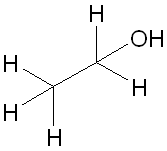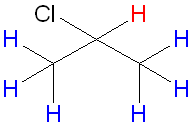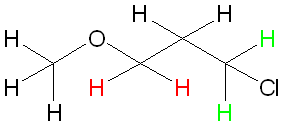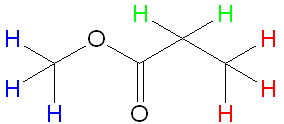# Draw structures for compounds that meet the following descriptions: a) C2H6O; one singlet b)...

## Question:

Draw structures for compounds that meet the following descriptions in proton NMR:

a) C2H6O; one singlet

b) C3H7Cl; one doublet and one septet

c) C4H8Cl2O; two triplets

d) C4H8O2; one singlet, one triplet, and one quartet

## Splitting Patterns in {eq}^1H {/eq}-NMR Spectroscopy

In {eq}^1H {/eq}-NMR spectroscopy, the signals from protons can be split according to the n+1 rule. This rule, where n is the number of protons adjacent to the proton giving the signal, tells us what the signal will split into. For example, if the proton has 3 adjacent protons, the signal will be split into 3+1=4 signals.

a) The structure that gives one singlet is shown below. The singlet is the O-H proton, since the oxygen shields the proton to couple with the adjacent protons.b) The structure that gives a septet and a doublet is shown below. The septet is given by the hydrogen in red, as there are 6 adjacent protons so n+1 gives 7. The doublet arises from the protons in blue. This is because these protons are in the same environment, so will give one peak. They are adjacent to one proton, so n+1 gives 2.c) The structure that gives two triplets is shown below. The triplet arises from the protons in green and red. This is because they are adjacent to only 2 protons, where n+1 gives 3. Additionally, they are not in the same environment, so we get 2 different signals.d) The structure that gives one singlet, one triplet and one quartet is given below. The singlet arises from the protons adjacent to the oxygen atom and are shown in blue. They have no adjacent protons and so their signal is not split. The triplet arises from the protons in red as there are two adjacent protons where n+1 gives 3. The quartet arises from the protons in green because they have 3 adjacent protons where n+1 is 4.